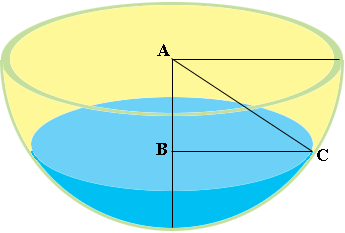SEARCH HOMEMath Central Quandaries & QueriesQuestion from Jimmy, a student: A bowl of water, being a hemisphere has a radius of 36cm. Water is filled in the bowl and has depth of 18 cm. Find the surface area of the water?Hi Jimmy,

I drew a diagram of your bowl.AB is a radius of the bowl so its length is 36 cm. The depth of the water is 18 cm so the length of AB is 18 cm. ABC is a right triangle. What does Pythagoras Theorem tell you about the length of BC?

PennyMath Central is supported by the University of Regina and The Pacific Institute for the Mathematical Sciences.